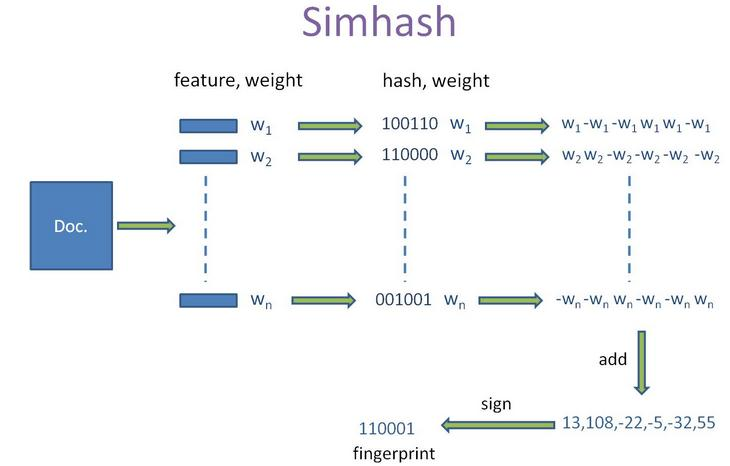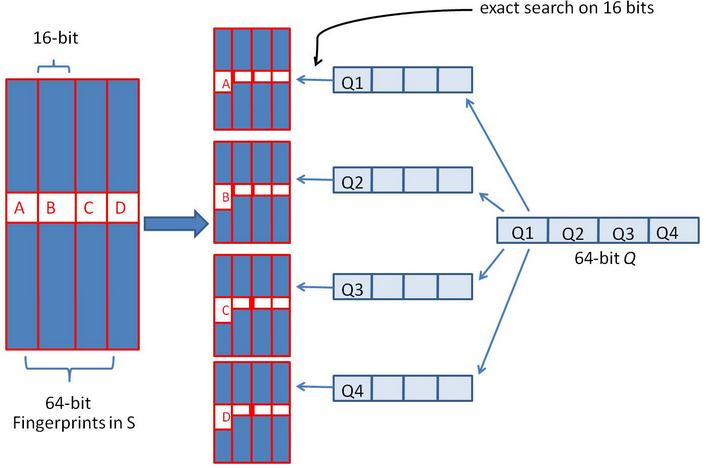http://gemantic.iteye.com/blog/1701101

cos或者MinHash算法

Simhash算法

simhash算法的输入是一个向量，输出是一个 f 位的签名值。为了陈述方便，假设输入的是一个文档的特征集合，每个特征有一定的权重。比如特征可以是文档中的词，其权重可以是这个词出现的次数。 simhash 算法如下：

1，将一个 f 维的向量 V 初始化为 0f 位的二进制数 S 初始化为 0

2，对每一个特征：用传统的 hash 算法对该特征产生一个 f 位的签名 b 。对 i=1f

3，如果 V 的第 i 个元素大于 0 ，则 S 的第 i 位为 1 ，否则为 0

4，输出 S 作为签名。对每篇文档根据SimHash 算出签名后，再计算两个签名的海明距离（两个二进制异或后 1 的个数）即可。根据经验值，对 64 位的 SimHash ，海明距离在 3 以内的可以认为相似度比较高。Java 代码实现：

1. package com.gemantic.nlp.commons.simhash;
2.
3. import java.math.BigInteger;
4. import java.util.ArrayList;
5. import java.util.List;
6. import java.util.StringTokenizer;
7.
8. public class SimHash {
9.
10.     private String tokens;
11.
12.     private BigInteger intSimHash;
13.
14.     private String strSimHash;
15.
16.     private int hashbits = 64;
17.
18.     public SimHash(String tokens) {
19.         this.tokens = tokens;
20.         this.intSimHash = this.simHash();
21.     }
22.
23.     public SimHash(String tokens, int hashbits) {
24.         this.tokens = tokens;
25.         this.hashbits = hashbits;
26.         this.intSimHash = this.simHash();
27.     }
28.
29.     public BigInteger simHash() {
30.         int[] v = new int[this.hashbits];
31.         StringTokenizer stringTokens = new StringTokenizer(this.tokens);
32.         while (stringTokens.hasMoreTokens()) {
33.             String temp = stringTokens.nextToken();
34.             BigInteger t = this.hash(temp);
35.             for (int i = 0; i < this.hashbits; i++) {
36.                 BigInteger bitmask = new BigInteger("1").shiftLeft(i);
37.                  if (t.and(bitmask).signum() != 0) {
38.                     v[i] += 1;
39.                 } else {
40.                     v[i] -= 1;
41.                 }
42.             }
43.         }
44.         BigInteger fingerprint = new BigInteger("0");
45.         StringBuffer simHashBuffer = new StringBuffer();
46.         for (int i = 0; i < this.hashbits; i++) {
47.             if (v[i] >= 0) {
49.                 simHashBuffer.append("1");
50.             }else{
51.                 simHashBuffer.append("0");
52.             }
53.         }
54.         this.strSimHash = simHashBuffer.toString();
55.         System.out.println(this.strSimHash + " length " + this.strSimHash.length());
56.         return fingerprint;
57.     }
58.
59.     private BigInteger hash(String source) {
60.         if (source == null || source.length() == 0) {
61.             return new BigInteger("0");
62.         } else {
63.             char[] sourceArray = source.toCharArray();
64.             BigInteger x = BigInteger.valueOf(((long) sourceArray) << 7);
65.             BigInteger m = new BigInteger("1000003");
66.             BigInteger mask = new BigInteger("2").pow(this.hashbits).subtract(
67.                     new BigInteger("1"));
68.             for (char item : sourceArray) {
69.                 BigInteger temp = BigInteger.valueOf((long) item);
71.             }
72.             x = x.xor(new BigInteger(String.valueOf(source.length())));
73.             if (x.equals(new BigInteger("-1"))) {
74.                 x = new BigInteger("-2");
75.             }
76.             return x;
77.         }
78.     }
79.
80.     /**
81.      * 取两个二进制的异或，统计为1的个数，就是海明距离
82.      * @param other
83.      * @return
84.      */
85.
86.     public int hammingDistance(SimHash other) {
87.
88.         BigInteger x = this.intSimHash.xor(other.intSimHash);
89.         int tot = 0;
90.
91.         //统计x中二进制位数为1的个数
92.         //我们想想，一个二进制数减去1，那么，从最后那个1（包括那个1）后面的数字全都反了，对吧，然后，n&(n-1)就相当于把后面的数字清0，
93.         //我们看n能做多少次这样的操作就OK了。
94.
95.          while (x.signum() != 0) {
96.             tot += 1;
97.             x = x.and(x.subtract(new BigInteger("1")));
98.         }
99.         return tot;
100.     }
101.
102.     /**
103.      * calculate Hamming Distance between two strings
104.      *  二进制怕有错，当成字符串，作一个，比较下结果
105.      * @author
106.      * @param str1 the 1st string
107.      * @param str2 the 2nd string
108.      * @return Hamming Distance between str1 and str2
109.      */
110.     public int getDistance(String str1, String str2) {
111.         int distance;
112.         if (str1.length() != str2.length()) {
113.             distance = -1;
114.         } else {
115.             distance = 0;
116.             for (int i = 0; i < str1.length(); i++) {
117.                 if (str1.charAt(i) != str2.charAt(i)) {
118.                     distance++;
119.                 }
120.             }
121.         }
122.         return distance;
123.     }
124.
125.     /**
126.      * 如果海明距离取3，则分成四块，并得到每一块的bigInteger值 ，作为索引值使用
127.      * @param simHash
128.      * @param distance
129.      * @return
130.      */
131.     public List<BigInteger> subByDistance(SimHash simHash, int distance){
132.         int numEach = this.hashbits/(distance+1);
133.         List<BigInteger> characters = new ArrayList();
134.
135.         StringBuffer buffer = new StringBuffer();
136.
137.         int k = 0;
138.         for( int i = 0; i < this.intSimHash.bitLength(); i++){
139.             boolean sr = simHash.intSimHash.testBit(i);
140.
141.             if(sr){
142.                 buffer.append("1");
143.             }
144.             else{
145.                 buffer.append("0");
146.             }
147.
148.             if( (i+1)%numEach == 0 ){
149.                 BigInteger eachValue = new BigInteger(buffer.toString(),2);
150.                 System.out.println("----" +eachValue );
151.                 buffer.delete(0, buffer.length());
153.             }
154.         }
155.
156.         return characters;
157.     }
158.
159.     public static void main(String[] args) {
160.         String s = "This is a test string for testing";
161.
162.         SimHash hash1 = new SimHash(s, 64);
163.         System.out.println(hash1.intSimHash + "  " + hash1.intSimHash.bitLength());
164.
165.         hash1.subByDistance(hash1, 3);
166.
167.         System.out.println("\n");
168.         s = "This is a test string for testing, This is a test string for testing abcdef";
169.         SimHash hash2 = new SimHash(s, 64);
170.         System.out.println(hash2.intSimHash+ "  " + hash2.intSimHash.bitCount());
171.         hash1.subByDistance(hash2, 3);
172.         s = "This is a test string for testing als";
173.         SimHash hash3 = new SimHash(s, 64);
174.         System.out.println(hash3.intSimHash+ "  " + hash3.intSimHash.bitCount());
175.         hash1.subByDistance(hash3, 3);
176.         System.out.println("============================");
177.         int dis = hash1.getDistance(hash1.strSimHash,hash2.strSimHash);
178.
179.         System.out.println(hash1.hammingDistance(hash2) + " "+ dis);
180.
181.         int dis2 = hash1.getDistance(hash1.strSimHash,hash3.strSimHash);
182.
183.         System.out.println(hash1.hammingDistance(hash3) + " " + dis2);
184.
185.
186.
187.     }
188. }

posted on 2015-04-17 14:43 SIMONE 阅读(478) 评论(0)  编辑  收藏 所属分类: JAVA只有注册用户登录后才能发表评论。 网站导航: 相关文章: